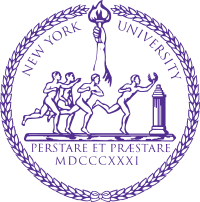## Columbia / Courant Joint Probability Seminar

### Columbia University, Wednesday April 19, 2017Speakers

Schedule

 4:00-4:30 pm Coffee and tea (Room 508) 4:30-5:30 pm Jean Bertoin (departmental colloquium) [A probabilistic approach to spectral analysis of growth-fragmentation equations] 5:45-6:30 pm Zhou Fan [Probabilistic analysis of a semidefinite program on sparse Erdos-Renyi graphs] 6:30-7:15 pm Cyril Labbé [Localisation of the continuous Anderson Hamiltonian in 1d]

Practical Information
The talks will take place at Columbia University Mathematics building in Room 520, on April 19, 2017. No registration necessary. However, if you would like to attend the group dinner after the talks, please RSVP.

Directions to Columbia.

Titles and Abstracts

Jean Bertoin (Zurich)
A probabilistic approach to spectral analysis of growth-fragmentation equations

(based on a joint work with Alex Watson, Manchester University).
The growth-fragmentation equation describes a system of growing and dividing particles, and arises in models of cell division, protein polymerisation and even telecommunications protocols. Several important questions about the equation concern the asymptotic behaviour of solutions at large times: at what rate do they converge to zero or infinity, and what does the asymptotic profile of the solutions look like? Does the rescaled solution converge to its asymptotic profile at an exponential speed? These questions have traditionally been studied using analytic techniques such as entropy methods or splitting of operators. In this work, we present a probabilistic approach to the study of this asymptotic behaviour. We use a Feynman-Kac formula to relate the solution of the growth-fragmentation equation to the semigroup of a Markov process, and characterise the rate of decay or growth in terms of this process. We then identify the Malthus exponent and the asymptotic profile in terms of a related Markov process, and give a spectral interpretation in terms of the growth-fragmentation operator and its dual.

Zhou Fan (Stanford)
Probabilistic analysis of a semidefinite program on sparse Erdos-Renyi graphs

Abstract: Spectral algorithms are a powerful method for detecting low-rank structure in dense random matrices and random graphs. However, in certain problems involving sparse random graphs with bounded average vertex degree, a naive spectral analysis of the graph adjacency matrix fails to detect this structure. In this talk, I will discuss a semidefinite programming (SDP) approach to address this problem, which may be viewed both as imposing a delocalization constraint on the maximum eigenvalue problem and as a natural convex relaxation of minimum graph bisection. I will discuss probabilistic results that bound the value of this SDP for sparse Erdos-Renyi random graphs with fixed average vertex degree, as well as an extension of the lower bound to the two-group stochastic block model. Our upper bound uses a dual witness construction that is related to the non-backtracking matrix of the graph. Our lower bounds analyze the behavior of local algorithms, and in particular imply that such algorithms can approximately solve the SDP in the Erdos-Renyi setting.
This is joint work with Andrea Montanari.

Cyril Labbé (Paris Dauphine)
Localisation of the continuous Anderson Hamiltonian in 1d

Abstract: We consider the Anderson Hamiltonian with a white noise potential on a segment of length L and endowed with Dirichlet boundary conditions. We show that, as L goes to infinity, the (appropriately rescaled and shifted) eigenvalues converge to a Poisson point process on R with an explicit intensity, and that the eigenfunctions converge to Dirac masses located at iid uniform points. Furthermore, we show that the shape of every eigenfunction near its maximum is given by an explicit, deterministic function which does not depend on the corresponding eigenvalue. This is a joint work with Laure Dumaz (Paris-Dauphine).